# R for Biologists

A collection of episodes with videos, codes, and exercises for learning the basics of the R programming language through examples.

### Introduction to R

#### R and Unix#### Beginnings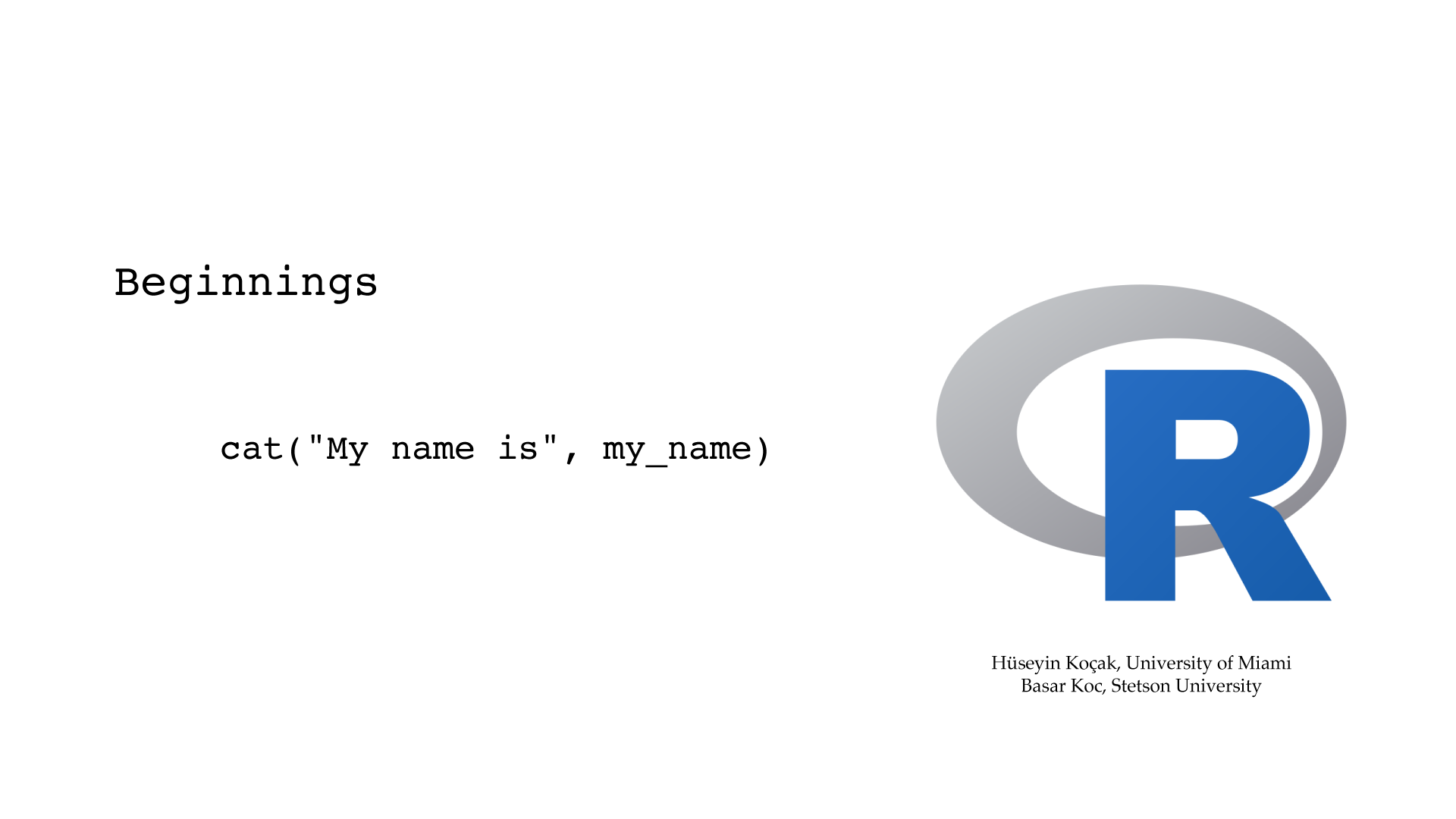```# ------------------------------------------------------------------
# File name: beginnings.R
#
# A line starting with # character is a comment line and is not interpreted by R
# Use comment lines liberally in your R scripts
# As you study these scripts, make sure to type each line in your copy of R
#
# Version: 2.1
# Authors: H. Kocak, University of Miami and B. Koc, Stetson University
# References:
# https://www.r-project.org
# ------------------------------------------------------------------

# R as a calculator
2+3.1

# Semicolon is a statement separator
2*3; 2/3

# R displays 7 digits by default. You can display more digits with options().
# More than 15 digits could be unreliable
# This is a global option; remains in effect until further notice
options(digits = 15)

# ^ for power
2/3; 2.1^3.1

# R has built-in mathematical constants and functions
2*pi
sin(2*pi)

# This is e
exp(1)

sqrt(2)

# Variables and their values are saved in the memory
# The assignment operators = or <- are equivalent. The right-hand-side
# is evaluated first and the result is assigned to the variable on the left.

# Variable names must start with a letter and can have letters, digits, underscore, and period.
# R distinguishes between upper and lower case
# Try to use suggestive, not cryptic, variable names

# x is a varible with value 2.1
x = 2.1

# To print the value of the variable x
x

# Same assignment as = in the previous statement
x <- 2.1
x

# plus_2 is another variable holding 2
plus_2 = 2.0

# Add the value of x and value of plus_2 and assign it as the new value of x
# old value of x is lost
x = x + plus_2

# Printing in R can be accomplished in three basic ways:

# simply type the variable name
x

# print(x) function: simply prints the value of x; its use sometimes necessary
# in loops and user-defined functions
print(x)

# cat() function concatenates strings, inside double quotes, and interpolated values of variables
cat("x =", x, "\n")
cat("root2 =", sqrt(2), "\n", "root3 =", sqrt(3), "\n")

# A variable can also hold a string in quotes
my_name = "Huseyin Kocak"
cat("My name is", my_name)

# You can quit R by
quit()

```

#### R Help```# ------------------------------------------------------------------
# File name: R_help.R
#
# R has readily accessible help facilities from the command line
# Below is an annotated summary of common help commands
# For more extensive information, see R Help
#
# Version: 2.1
# Authors: H. Kocak, University of Miami and B. Koc, Stetson University
# References:
# https://www.r-project.org
# ------------------------------------------------------------------

# To see a sequence of simple graphics pictures
demo(graphics)

# To see a sequence of 3D graphics
demo(persp)

# To list available demonstrations in base package of R
demo()

# To list all arithmetic operators in R
help(Arithmetic)

# To get help on the log function
help(log)

# To display R help on the plot() function
help(plot)

# Same as help(plot)
?plot

# To see example of the usage of plot function
example(plot)

# To list all R commands containing the string plot; could be too much info
help.search("plot")

# Same as help.search("plot")
??plot

# To get more help on the help() function
help()

```

#### Vectors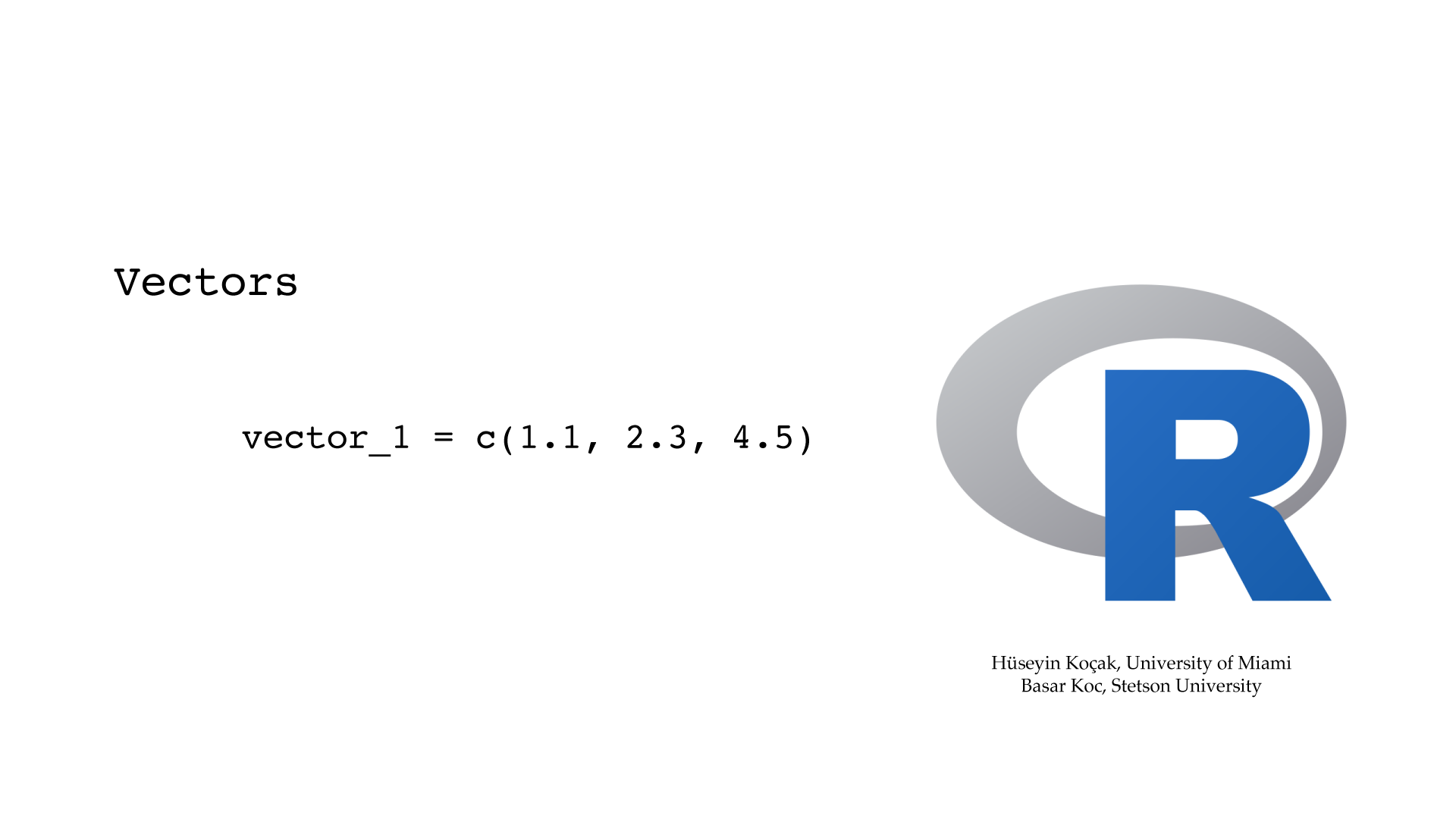```# ------------------------------------------------------------------
# File name: vectors.R
#
# R is efficient in computing with vector variables
# By default, a variable holding a single number is a vector of length 1
# Below is an annotated summary of common operations with vector variables
#
# Version: 2.1
# Authors: H. Kocak, University of Miami and B. Koc, Stetson University
# References:
# https://www.r-project.org
# ------------------------------------------------------------------

# R function c() combines (concatanes) values into a vector
x = c(1.5, -3.2, 0.45, 4.1, 10)
print(x)

# Entry of a vector can be addressed by its index, its position in the vector
# Unlike other programming languages, index of a vector in R starts from 1

# To print the first entry of x
print(x)

# To print the 4th entry of x
print(x)

# To change the value of the 4th entry of x to 7.6
x = 7.6
print(x)

# Can add an entry to a vector
x = 91
print(x)

# An entry of a vector can be deleted using -index
x = x[-6]
print (x)

# R function length() returns the number of entries of a vector
# There are numerous function for vectors; try, for example
# sort(), rev(), min(), mean(), sum(), prod()

length_of_x = length(x)
print(length_of_x)

# Arithmetic of vectors is done entry-wise
# + addition, - subtraction, * product, / division, %*% crossproduct

vector_1 = c(1.1, 2.3, 4.5)
vector_2 = c(-1, 1.2, 0.5)
vector_sum = vector_1 + vector_2
print(vector_sum)

# Vector entries can be strings or booleans
nucleotides = c("A", "C", "G", "T")
print(nucleotides)

# Vectors with patterns are useful. We will see a better way to generate them
x = c(0, 0.5, 1.0, 1.5, 2.0, 2.5, 3.0)
print (x)

squareRoot_x = sqrt(x)
print(squareRoot_x)

```

#### Sequences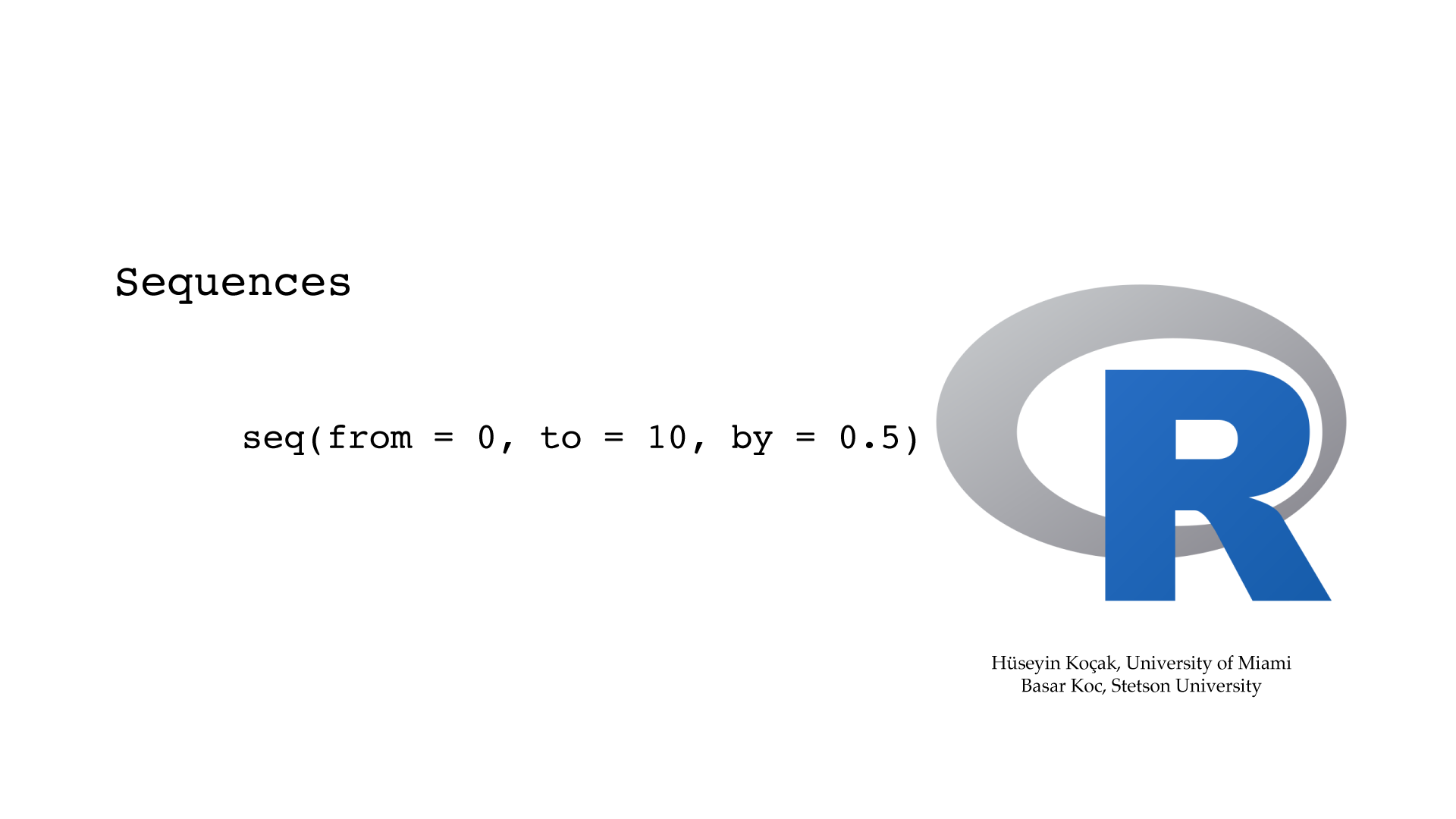```# ------------------------------------------------------------------
# File name: sequences.R
#
# seq() function is used to generate a vector with specified patterns
# Below are common usages of seq() and some shortcuts for generating sequences
#
# Version: 2.1
# Authors: H. Kocak, University of Miami and B. Koc, Stetson University
# References:
# https://www.r-project.org
# ------------------------------------------------------------------

# To generate a sequence with fixed increments and assign it to variable x
x = seq(from = 0, to = 10, by = 0.5)
print(x)

# The construction above can be shorten as
x = seq(0, 10, 0.5)
print(x)

# The same sequence above can be generated by specifying the number of entries
x = seq(from = 0, to = 10, length = 21)
print(x)

# To generate a sequence with increment 1 (by = 1) can be shorten as
x = seq(0, 10)
print(x)

# The sequence above can be shorten further as
x = 0:10
print(x)

# : operator has higher predecence than the arithmetical operations.
# Note the difference in the two sequences below

sequence_1 = 0 : 10 - 2
print(sequence_1)

sequence_2 = 0 : (10 - 2)
print(sequence_2)

```

#### Script```# ------------------------------------------------------------------
# File name: script.R
#
# A simple R script to plot square root function
# The graph will be output to the screen in a separate graphics window
#
# To execute R commands in a file, type source("filename.R") at the propmt
# R looks for files in a "working directory"; type getwd() to find out this directory
# Use setwd() to set working directory. For example, if the file script_plot.R is in the
# /Users/hk/Documents/R_scripts directory, type
# setwd("/Users/hk/Documents/R_scripts/") then source("script.R")
# In Unix, you can start R from the directory containing your R scripts
#
# Version: 2.1
# Authors: H. Kocak, University of Miami and B. Koc, Stetson University
# References:
# https://www.r-project.org
# ------------------------------------------------------------------

x = seq(from = 0, to = 10, by = 0.5)
square_root_x = sqrt(x)

plot(x, square_root_x)

```

1. Multiplication table: Write an R code that prints out the 9x9 multiplication table. For this problem, you need to create a variable that holds the sequence of integers from 1 to 9. By multiplying this sequence repeatedly with appropriate numbers, you can print out the multiplication table.

2. Vectors: Write an R code that prints out the odd numbers from 1 to 13. Use the R function sum() to compute the sum of these odd numbers. Use the R function mean() to compute the mean of these odd numbers. You should use the R function cat() to get a nice output.

3. Random numbers: Random numbers with desired distributions (e.g. uniform or normal) are important in statistical data analysis. Look up the descriptions of R functions runif(), rnorm(), mean(), and sd() using the help facilities of R.

The R function runif(10, min=0, max=1) generates 10 random numbers uniformly distributed between 0 and 1. Generate 1000 random numbers uniformly distributed between 0 and 3 and save them in a vector. Compute the mean of these numbers. Is the computed value of the mean acceptable?

The R function rnorm(10, mean=0,sd=1) generates 10 random numbers with mean = 0 and standard deviation = 1. Generate 1000 random numbers with mean = 150, sd = 9 and save them in a vector. Compute the mean and the standard deviation of these numbers. Are the computed values of these two numbers acceptable?

### Graphics

#### Plot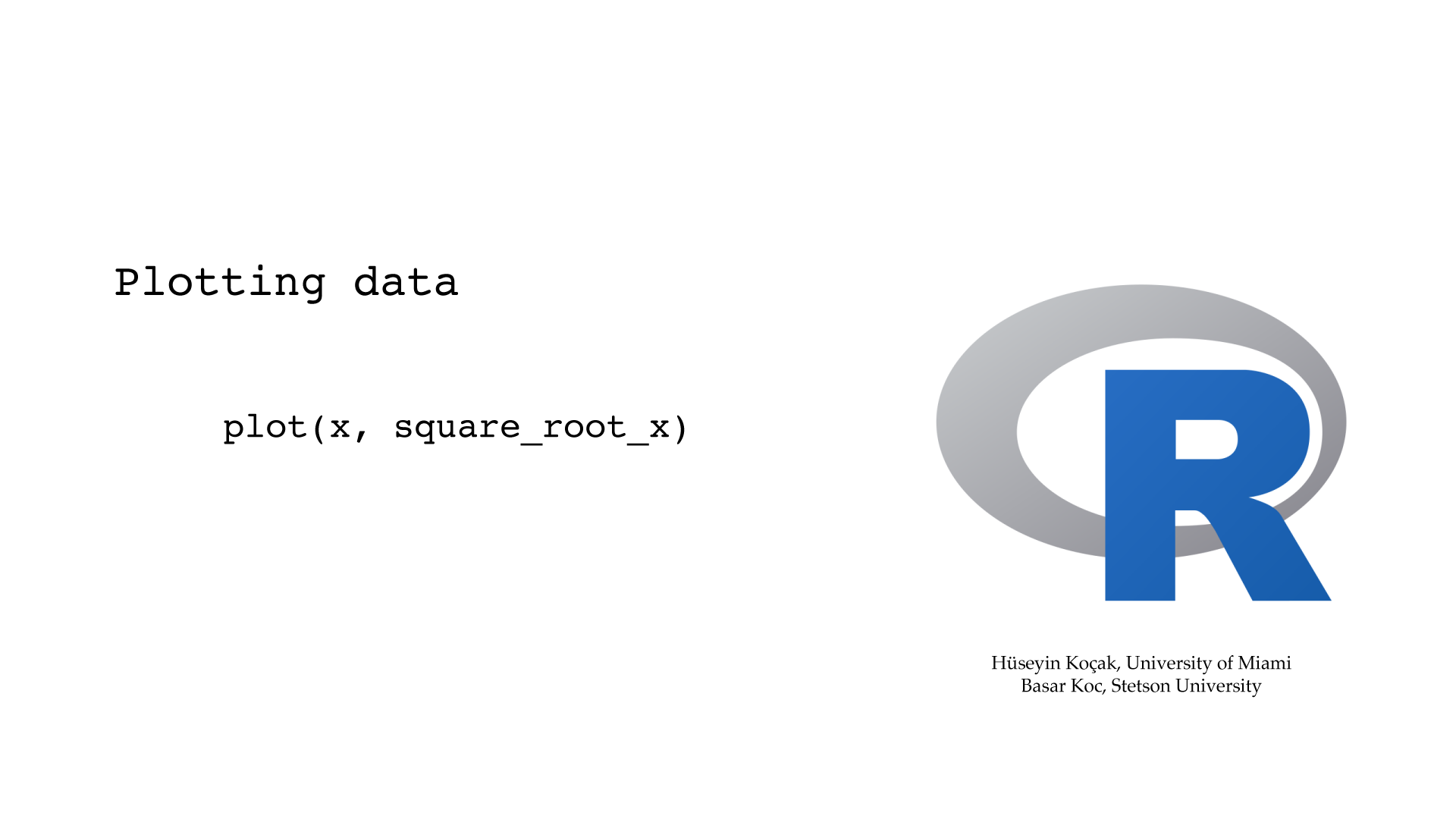```# ------------------------------------------------------------------
# File name: plot_options.R
#
# plot() is a high-level R function that opens a new plotting window
# Here, we will show some basic options to plot() with a sequence of plots
# of square root function with increasing complexity
# Type ?plot to see details of basic options; you can see
# 657 available colores by typing colors()
#
# Version: 2.1
# Authors: H. Kocak, University of Miami and B. Koc, Stetson University
# References:
# https://www.r-project.org
# ------------------------------------------------------------------

x = seq(from = 0, to = 10, by = 0.5)
square_root_x = sqrt(x)

# Plot x-values vs. square_root_x values with default options
plot(x, square_root_x)

# To pause between plots

plot(x, square_root_x, main = "My First Plot")

# To add label to y-axis
plot(x, square_root_x, main = "My First Plot", ylab = "sqrt(x)")

# To set limits of y-axis
plot(x, square_root_x, main = "My First Plot", ylab = "sqrt(x)", ylim = c(0, 10))

plot(x, square_root_x, main = "My First Plot", ylab = "sqrt(x)", ylim = c(0, 10),
col = "blue")

# To over strike with both plotting characters, pch, and connecting lines
plot(x, square_root_x, main = "My First Plot", ylab = "sqrt(x)", ylim = c(0, 10),
col = "blue", type = "o")

# To set the line type
plot(x, square_root_x, main = "My First Plot", ylab = "sqrt(x)", ylim = c(0, 10),
col = "blue", type = "o", lty = "dotted")

# To set plotting character
plot(x, square_root_x, main = "My First Plot", ylab = "sqrt(x)", ylim = c(0, 10),
col = "blue", type = "o", lty = "dotted", pch = 22)

```

#### Saving To File```# ------------------------------------------------------------------
# File name: saving_to_file.R
#
# Saving the plot of square root function to a png file
# You can also save a picture as a pdf file by using the
# pdf() function with .pdf file extension
#
# The file is saved in the current working directory of R
# Existing file with same name is overwritten
# The graph will not be output to the screen, so you should save
# after you get the desired picture
#
# Version: 2.1
# Authors: H. Kocak, University of Miami and B. Koc, Stetson University
# References:
# https://www.r-project.org
# ------------------------------------------------------------------

png("square_root_function.png", bg = "gray", width = 600, height = 600)

x = seq(from = 0, to = 10, by = 0.5)
square_root_x = sqrt(x)

plot(x, square_root_x, main ="Graph of Square root function", xlim = c(0, 10), ylim = c(0, 10),
col = "red", type = "o")

dev.off()

```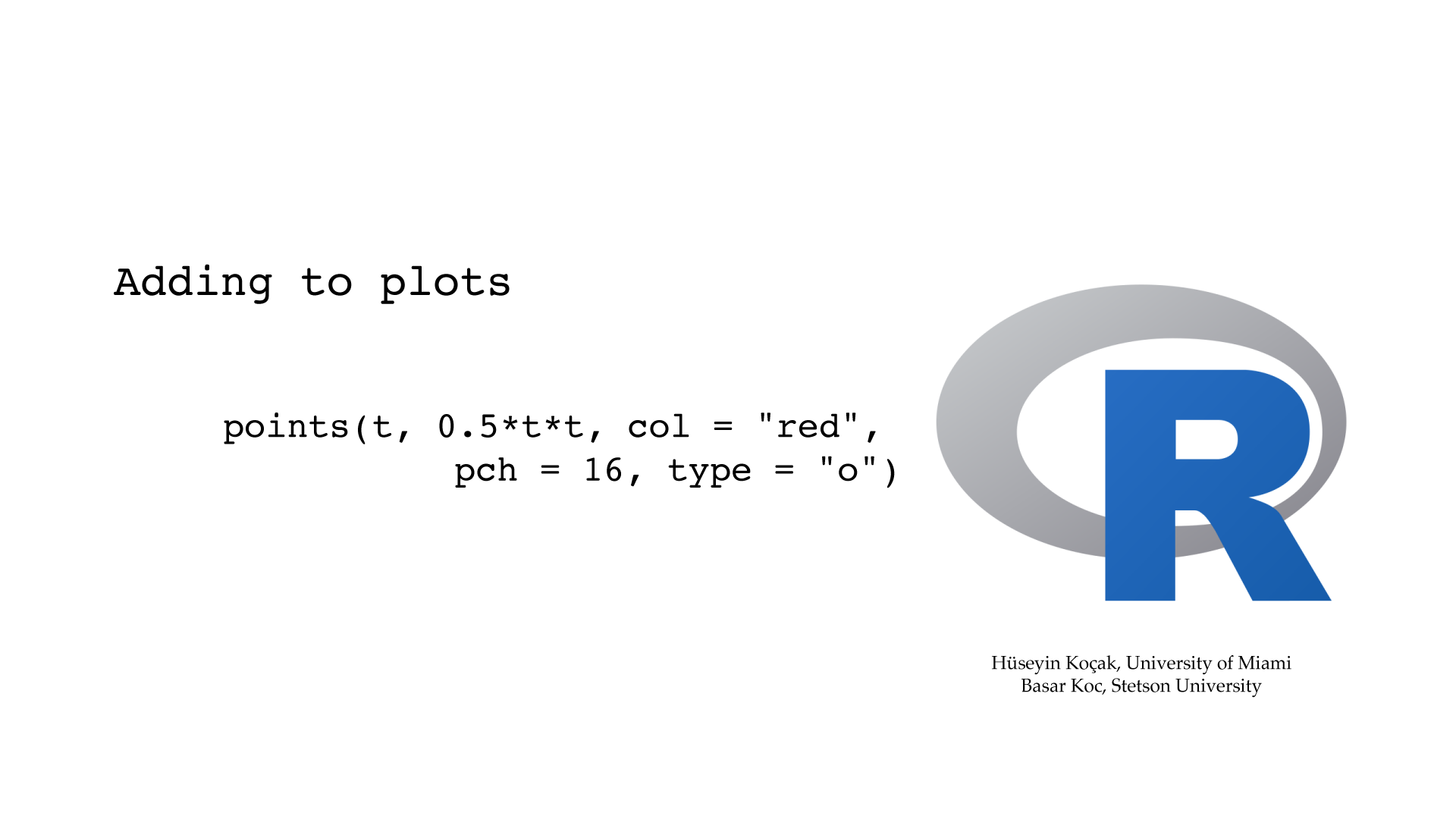```# ------------------------------------------------------------------
#
# plot() is a high-level plotting function that opens a new plotting window
# using low-level functions, one can add to the open plot
# some of the commonly used adding functions are:
# points(), lines(), abline(), legend(), text()
# Below, we will plot a function and add to plot
# its inverse function, a line, text, and a legend
#
# Version: 2.1
# Authors: H. Kocak, University of Miami and B. Koc, Stetson University
# References:
# https://www.r-project.org
# ------------------------------------------------------------------

t = seq(from = 0, to = 10, by = 0.5)

# To pause between plots

plot (t, sqrt(2 * t), main = "Adding to Plot", sub = "A function and its inverse",
xlab = "", ylab = "", xlim = c(0, 10), ylim = c(0, 10), pch = 16, type = "o")

# To add the inverse function
points(t, 0.5 * t * t, col = "red" , pch = 16, type = "o")

# To add the line with intercept = 0, slope = 1
abline(0, 1, col = "gray", lwd = 3, lty = "dashed")

text(7, 7.3, "45-degree line", srt = 45)
legend("bottomright", legend = c("funtion", "inverse"), pch = 16, col = c("black", "red"))

# One can add graphs of mathematical functions using, for example:
# lines(curve(sin(x) + cos(x) + 5,  add = TRUE))

```

#### Curve Plotting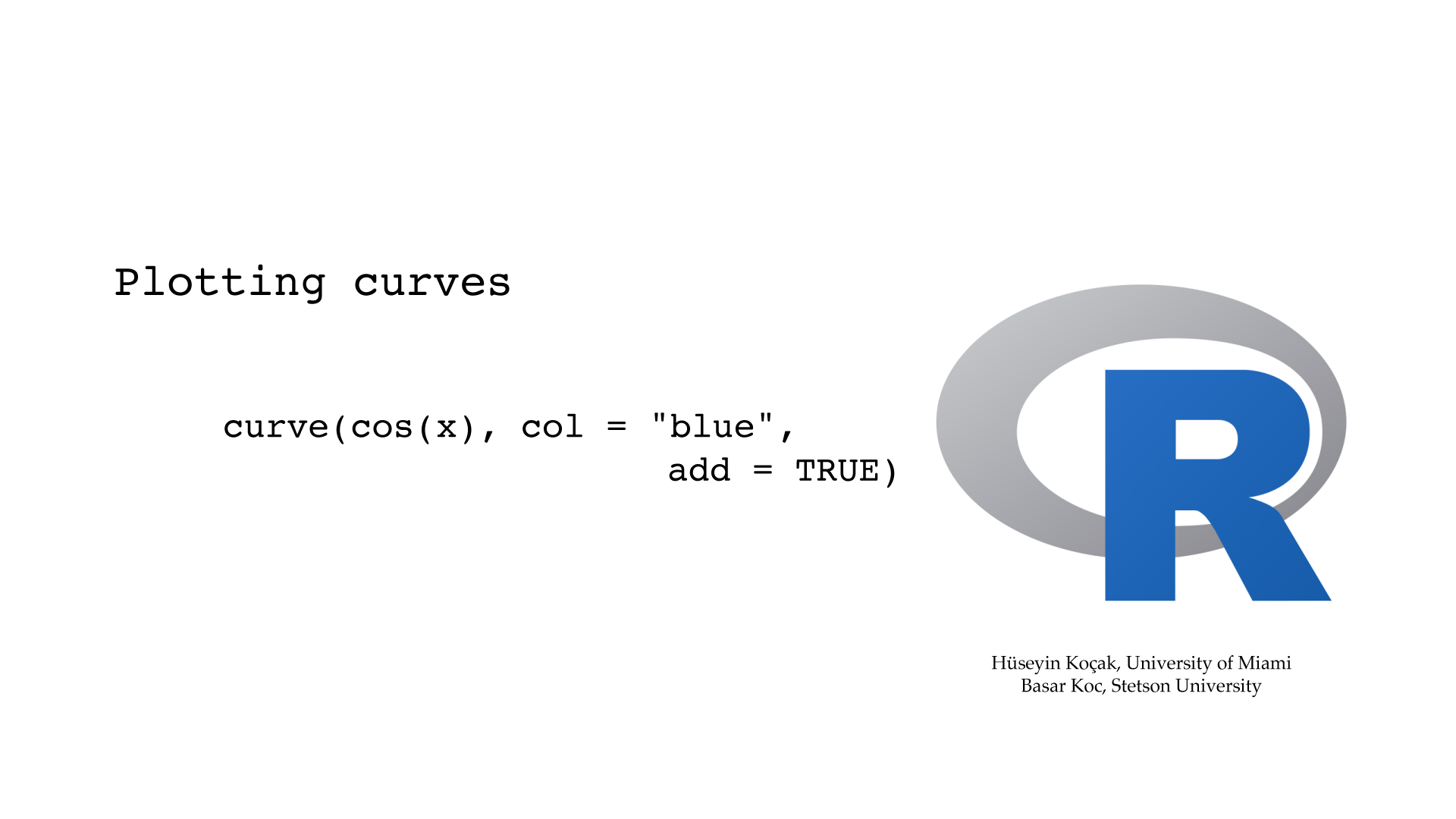```# ------------------------------------------------------------------
# File name: curve_plotting.R
#
# curve() is a high-level mathematical plotting function that opens a new plotting window
# Variable in the mathematical formula must be x
#
# Version: 2.1
# Authors: H. Kocak, University of Miami and B. Koc, Stetson University
# References:
# https://www.r-project.org
# ------------------------------------------------------------------

curve(sin(x), xlim = c(-4*pi, 4*pi), ylim = c(-2, 2), col = "red", ylab = "")

# To plot horizontal line using h
abline(h=0, lty= "dotted")

# To mark the origin
points(0, 0, pch = 3)

curve(cos(x),  col = "blue", add = TRUE)
curve(sin(x) + cos(x),  col = "purple", lwd = 3, add = TRUE)

title("sin(x) + cos(x)")

legend("bottomright", legend = c("sin(x)", "cos(x)", "sin(x) + cos(x)"),
lty = 1, col = c("red", "blue", "purple"))

```

#### Multiple Plots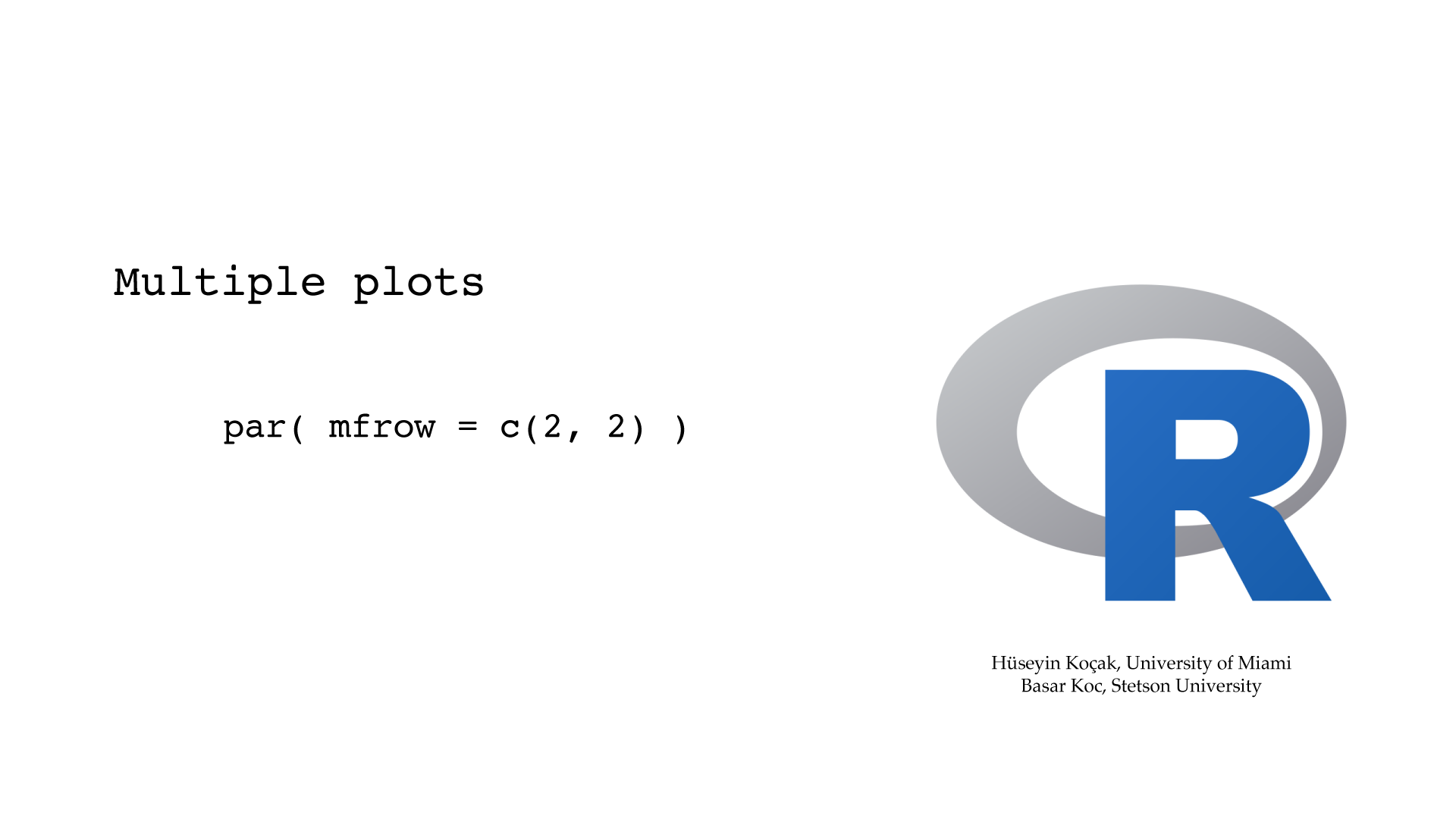```# ------------------------------------------------------------------
# File name: multiple_plots.R
#
# With the par() command can set the multi plot environment.
# mfcol = c(nr,nc) partitions the graphic window as a matrix of nr rows and nc columns,
# the plots are then drawn in columns.
# mfrow = c(nr,nc) partitions the graphic window as a matrix of nr rows and nc columns,
# the plots are then drawn in rows.
# You can get fancy partitions of the plotting window with layout() function
#
# Version: 2.1
# Authors: H. Kocak, University of Miami and B. Koc, Stetson University
# References:
# https://www.r-project.org
# ------------------------------------------------------------------

# Prepare for 2x2 plots, to be filled by rows
par(mfrow = c(2, 2))

t = seq(0, 10, 0.2)
plot(t, sin(t))
curve(sinh(x), -5, 5)
curve(tan(x), n = 500, -5, 5)
curve(round(x), n = 500, -5, 5)

par(mfrow = c(1, 1))

curve(sin(x), -5, 5)

```

1. Heating of a probe: A student held a temperature probe between her two fingers and recorded the following temperatures every 2 seconds:
Time     Temp
00     32.77
02     33.16
04     33.35
06     33.57
08     33.67
10     33.75
12     33.85
14     33.94
16     33.98
18     34.03
20     34.04

Plot the temps as a function of time. Is the temp leveling off? Why?
2. Reset the clock: Copy the R code below and run (source) it. Modify the R file so that the clock shows 3:30. Include a picture of your new clock and the source file. For this task, look up in the R manual how arrows() function works, and also how to output your picture to a file format (pdf, png, ...) of your choice.
Extra credit: Can you set the clock to any prescribed time XY:WZ (hour:minute)?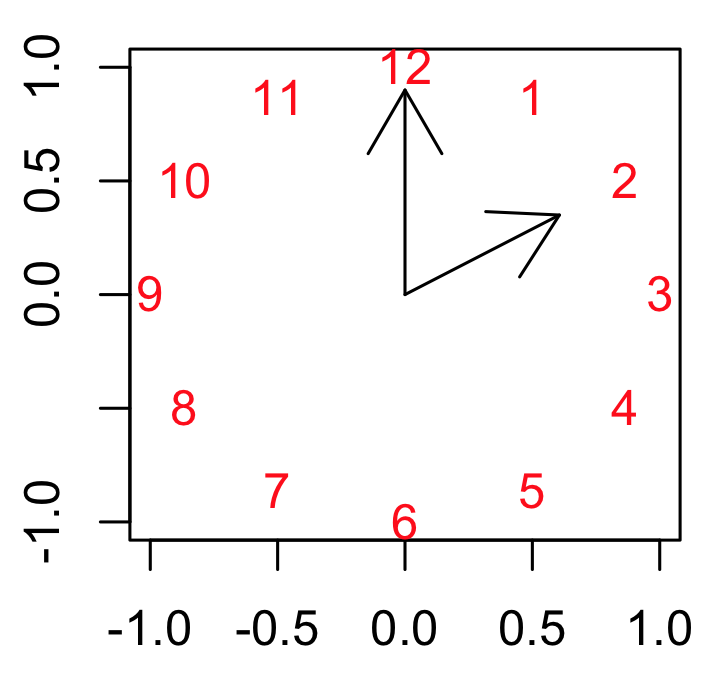3. ```# clock by Hlynka
# Look up in the R manual the function arrows()

plot(-1:1, -1:1,  type = "n",  xlab = "",  ylab = "" )
K = 12
text(exp(1i * 2 * pi * c(2:1,12:3) / K),  col = "red")
arrows( 0, 0, 0, 0.9)
arrows( 0, 0, 0.7*cos(pi/6), 0.7*sin(pi/6))
```

4. Energy conserved: The Energy of planar pendulum without friction is given by the function
E(Θ , Θ') = (1/2)m(L^2)(Θ'^2) + mgL(1- cos(Θ)).
This Energy function is supposed to be conserved along the solutions of the pendulum. Show this assertion by computing dE/dt using the chain rule, keeping in mind that Θ and Θ' depend on t, and the differential equations for pendulum. Observe that dE/dt = 0, so it must be constant function of t.

5. Free energy from EULER: Now, in the pendulum equation, take c= 0, m=1.3, l=1.25, g=1, the initial conditions Θ=0.6 and Θ'=0 and compute the solution for 20 time units using Euler's algorithm with step size of h=0.2. On the Time Panel of Phaser's Numerics Editor set "Skip iterations per plot" to 9 so that in the Xi Values view you will get the solution tabulated at even integer values of time: you should have 11 rows of numbers. Now, with R, use these position and velocity numbers in the formula for the Energy of the pendulum above so that you will have 11 values of the Energy function. Plot these Energy values as a function of time. Are these Energy values constant? Increasing? Decreasing?

6. Free energy from Runga-Kutta(4)? Repeat the problem above using Runga-Kutta(4), leaving all the other settings the same. Are the energy values constant? Increasing? Decreasing?

### Conditionals and Loops

#### If Else```# ------------------------------------------------------------------
# File name: if_else.R
#
# This code asks the user to enter a number.
# If the number is non-negative, it prints the sqrt of the number;
# otherwise, it exits with a message.
# Decision is accomplished with an if() else statement
#
# Version: 2.1
# Authors: H. Kocak, University of Miami and B. Koc, Stetson University
# References:
# https://www.r-project.org
# ------------------------------------------------------------------

cat("Please enter a number to compute its square root \n")

# To get input from keyboard (single item)
x = scan(nmax = 1, quiet = TRUE)

cat("The number you have entered is", x, "\n")

# Else must be on the same line as the closing brace of if
if(x >= 0) {
cat("square root of", x, "is", sqrt(x), "\n")
} else {
cat("Sorry, please enter a nonnegative number \n")
}

cat("Bye!. \n")

```

#### For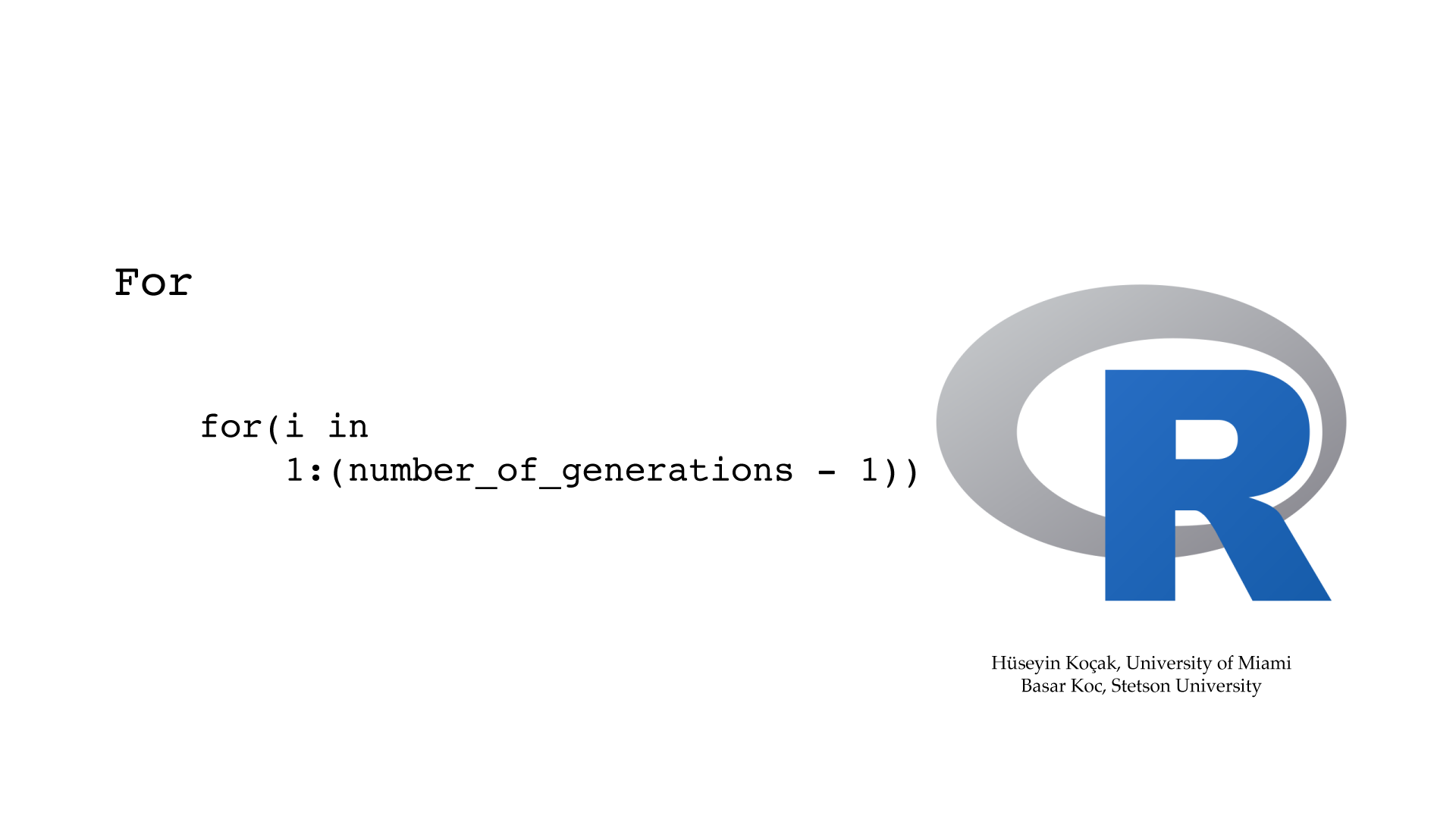```# ------------------------------------------------------------------
# File name: for_loops.R
#
# for loop in R iterates a block of statements with a counter running through
# values in a sequence. Syntax of for loop is:
# for (counter in sequence) {
#	statements
# }
#
# Version: 2.1
# Authors: H. Kocak, University of Miami and B. Koc, Stetson University
# References:
# https://www.r-project.org
# ------------------------------------------------------------------

sequence = seq (1, 10)

for(counter in sequence) {
squared = counter * counter
cat("counter = ", counter, "\t", "squared = ", squared, "\n" )
}

# In the following more complicated example of for loop, we will compute 10 generations of
# a population growing according to the discrete logistic model and save the
# population densities as a vector

cat("Densities of generations with discrete logistic growth model \n")

# Initialize vector holding population densities; x = 0.2
x = 0.2

cat("generation =", 1, "\t", "population density =", x, "\n" )

# Growth rate
r = 2.45
number_of_generations = 10

for(i in 1:(number_of_generations - 1)) {
x[i+1] = r*x[i] * (1.0 - x[i])
cat("generation =", i+1, "\t", "population density =", x[i+1], "\n" )
}

# To see x as a vector
print (x)

```

#### While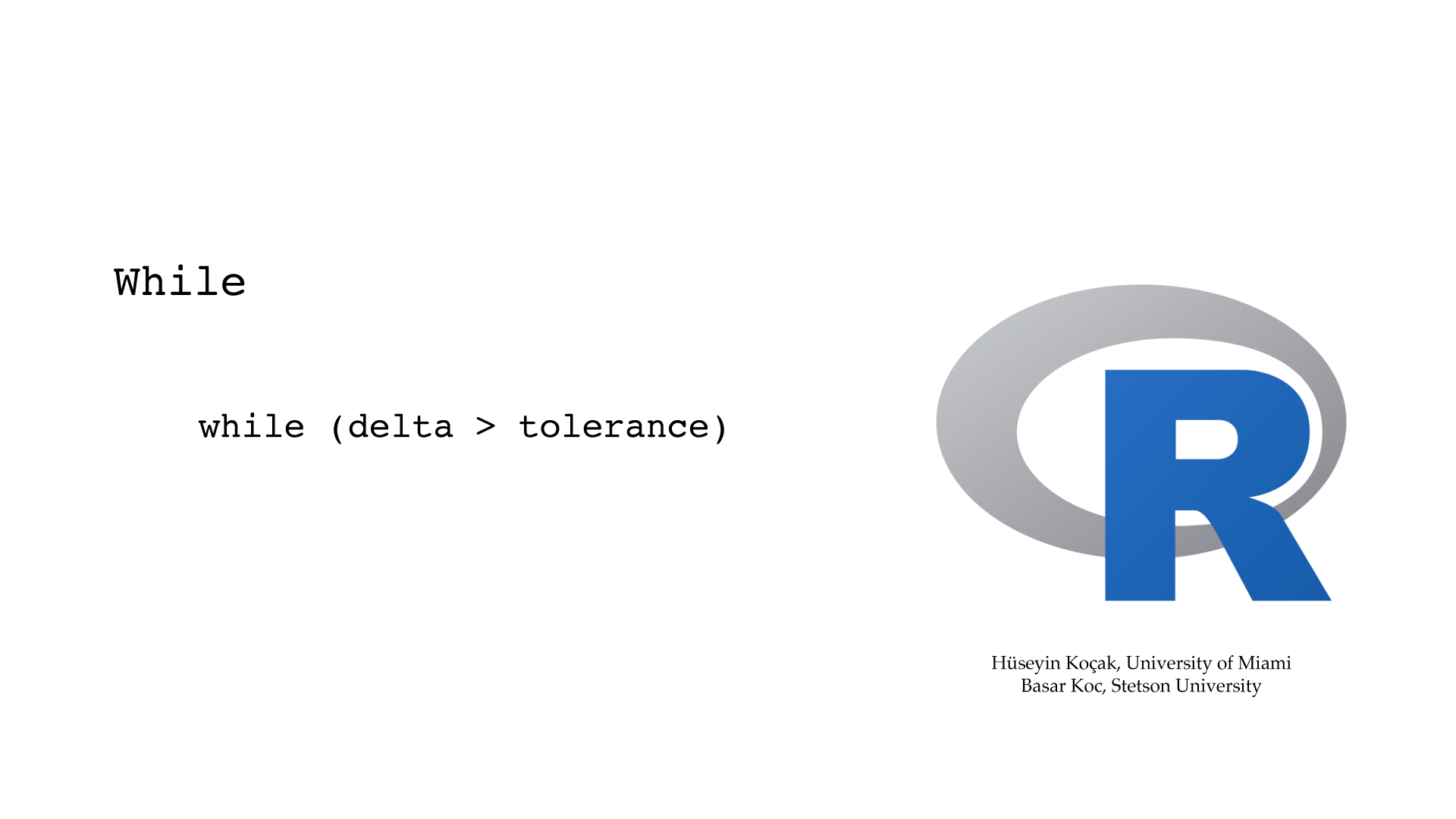```# ------------------------------------------------------------------
# File name: while_loops.R
#
# while loop iterates a block of statements as long as a condition (boolean) remains true.
# Syntax of while loop is:
# while (boolean){
#	statements
# }
# break exits the loop
#
# Version: 2.1
# Authors: H. Kocak, University of Miami and B. Koc, Stetson University
# References:
# https://www.r-project.org
# ------------------------------------------------------------------

# To generate a table of numbers and their squares

counter = 1

while (counter <= 10){
square = counter * counter
cat("counter = ", counter, "\t", "square = ", square, "\n" )
counter = counter + 1
}

# Newton-Raphson iteration for finding sqrt(2) with a specified tolerance
# x = starting value
# delta = absolute value of the difference between two consecutive iterates
# while performs Newton-Raphson iterates as long as delta remains greater than
# a desired tolerance

cat("Newton-Raphson iteration for computing sqrt(2) with a specified tolerance \n")
options(digits = 15)

x = 3.1
tolerance = 1e-8
cat("x =", x, "\t", "tolerance =", tolerance, "\n")

x_next = 0.5 * (x + 2/x)
delta = abs(x_next - x)
cat("x =", x_next, "\t", "delta =", delta, "\n")
x = x_next

while (delta > tolerance){
x_next = 0.5 * (x + 2/x)
delta = abs(x_next - x)
cat("x =", x_next, "\t", "delta =", delta, "\n")
x = x_next
}

```

1. Multiplication table: Write an R code that prints out the 9x9 multiplication table. For this problem, you need to use two for statements, one inside the other. Separate the entries on a line of the table with tabs.

2. Logistic XivsTime plot: Please write an R code to recreate the picture below. Use a for loop to iterate with the logistic map with the indicated initial condition and parameter. In plotting, make sure to get the initial point right.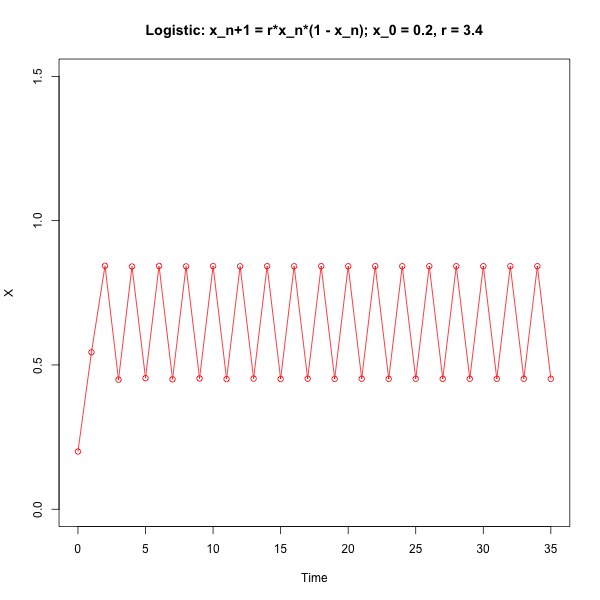3. Computing with Euler: Write an R code that computes the solution of the frictionless planar pendulum with Euler's algorithm. Take step_size = 0.1, number_of_steps = 200. Set the parameters in the pendulum as g = 1, L = 1.3, initial_position = 0.7, initial_velocity = 0.0. Plot the position and the angular velocity of the pendulum vs. time, as shown in the figure below. Note: after you plot the position variable with plot(...), you can add the velocity using points(...) function of R. You might look at some of the sample R codes below.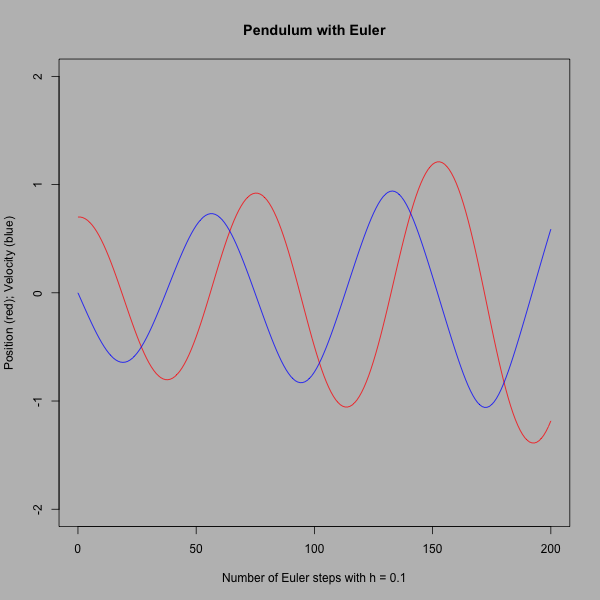4. Chaotic Logistic map as a pseudo random number generator: In the early days of search for pseudo random number generators, the chaotic dynamics of the Logistic map xn+1 = r * xn * (1.0 - xn) for the parameter value r = 4.0 was one of the candidates at Los Alamos. The picture below shows a comparison of the histograms of the 3000 iterates of the initial value x0 = 0.2 under the Logistic map, and the pseudo random number generator of the R function runif(3000).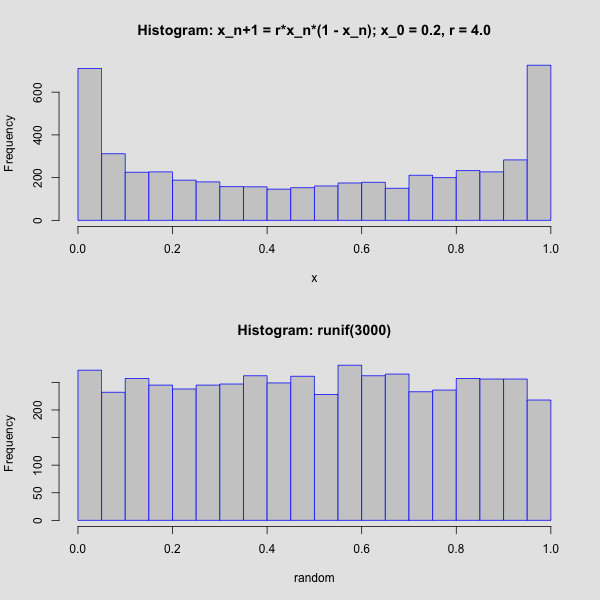• Consult R help for information, and the options, about runif() and hist() functions.
• Write an R script to reconstruct the picture above. Here, the number of bins is set to 20 by using the breaks = 20 option in hist().
• Experiment with increasing the number of iterates and the number of bins.
• Argue for or against using the chaotic Logistic map as a pseudo random number generator.

### Writing Functions

#### Writing Functions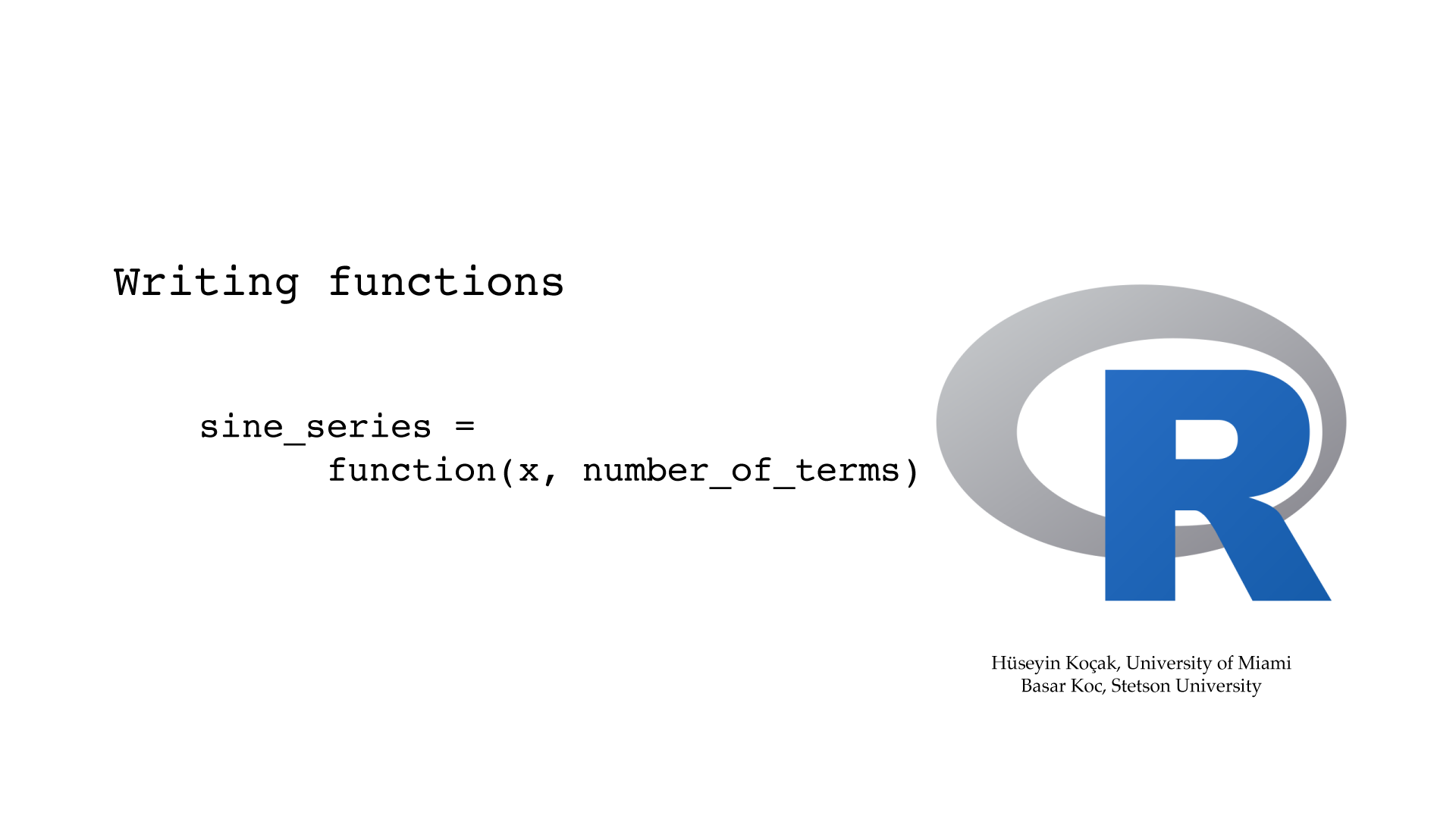```# ------------------------------------------------------------------
# File name: writing_functions.R
#
# User-defined functions have the form
# name = function(parameters ){
#	statements
# return()
# }
# After sourcing this file, the functions herein will be loaded into memory.
# The second function computes and returns the Taylor series approximation of sin(x) to
# a specified number of terms. Usage: sine_series(0.5, 5) to compute the
# sin of 0.5 for 5 terms.
#
# Version: 2.1
# Authors: H. Kocak, University of Miami and B. Koc, Stetson University
# References:
# https://www.r-project.org
# ------------------------------------------------------------------

cat("Huseyin Kocak\n")
cat("Department of Computer Science\n")
cat("University of Miami\n")
cat("Coral Gables, FL 33146\n")
}

options(digits = 15)

sine_series = function(x, number_of_terms){
sum = 0

for(n in 0:(number_of_terms-1)){
sum = sum + ( ((-1)^n) / factorial(2 * n + 1) ) * (x^(2 * n + 1) )
}

return(sum)
}

```

1. The R function below computes the square root of 2 using Newton iteration with an initial guess of x for number_of_iterations. Modify this function as
```newtonFor_A = function(A, x, number_of_iterations)
```
to compute the square root of any positive number A.
2. ```#newton iteration for finding sqrt(2)
#parameters
# x = starting value
# number_of_iterations = number of iterations
#usage: newtonFor(3.1, 8)

#to get more digits use options(digits=15)

newtonFor = function(x, number_of_iterations){  #begin function
print(x)
for ( i in 1:number_of_iterations){    #index i will run from 1 to number_of_iterations
x = 0.5*(x + 2/x)                    #replace old x with new value
print (x)
}                                      #end for
}                                          #end function
```
3. Ricker Model: The R code below computes and plots the discrete population model of Ricker for three parameter values. Modify this code to add to the plot a forth one for the parameter value r = 3.5.
4. ```# This script is from R for Beginners, by E. Paradis.
# ricker function iterates the Ricker model, a difference equation for
# modeling the growth of a single population, and plots fifty generations
# for three values of parameter r.

ricker <- function(nzero, r, K=1, time=50, from=0, to=time)
{
N=0
N <- nzero
for (i in 1:time) N[i+1] <- N[i]*exp(r*(1 - N[i]/K))
Time <- 0:time
plot(Time, N, type="o", xlim=c(from, to))
}

layout(matrix(1:3, 3, 1))
ricker(0.1, 1); title("Ricker model with r = 1")
ricker(0.1, 2); title("r = 2")
ricker(0.1, 3); title("r = 3")

```

### Curve Fitting and Parameters

#### Curve Fitting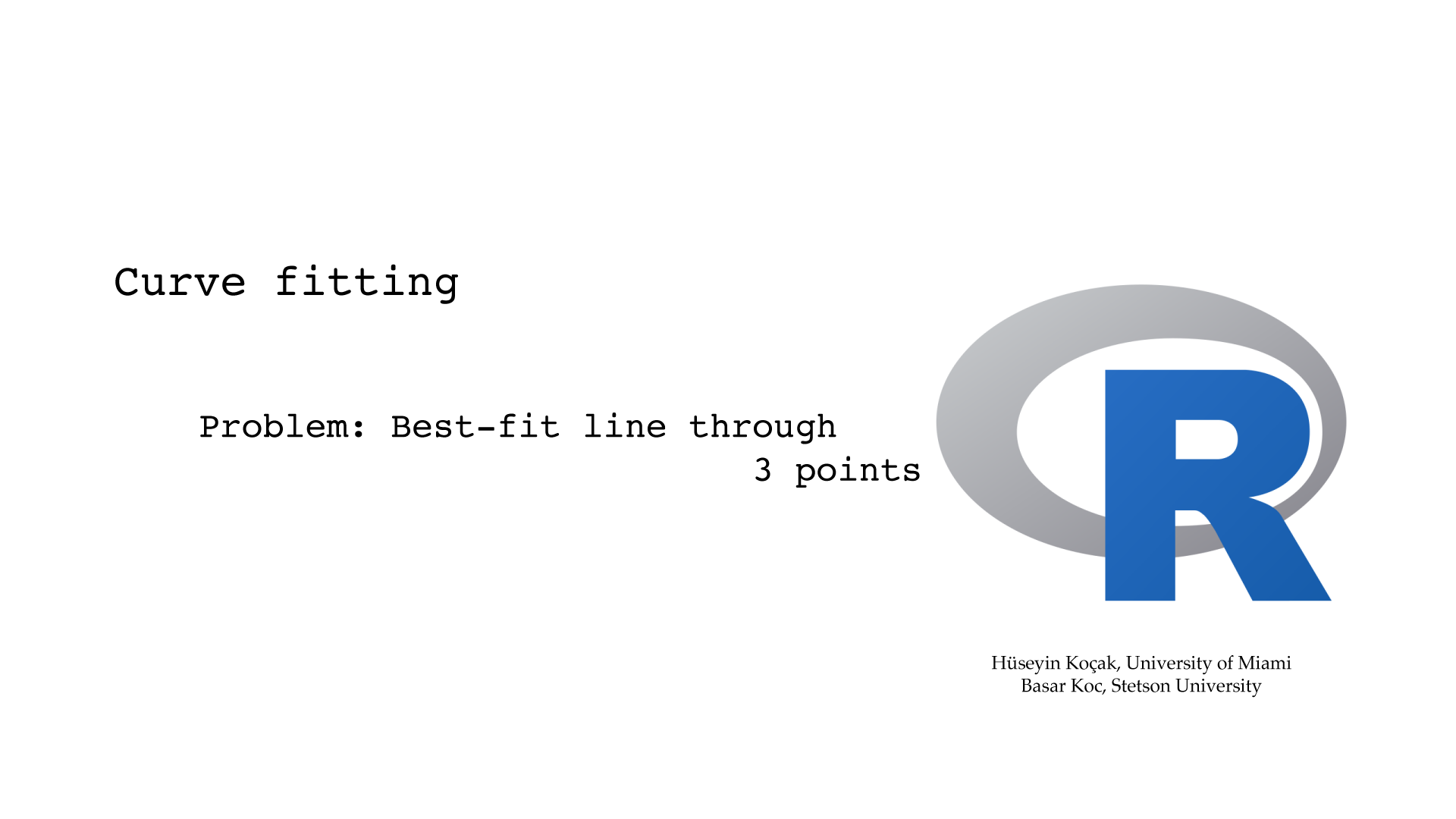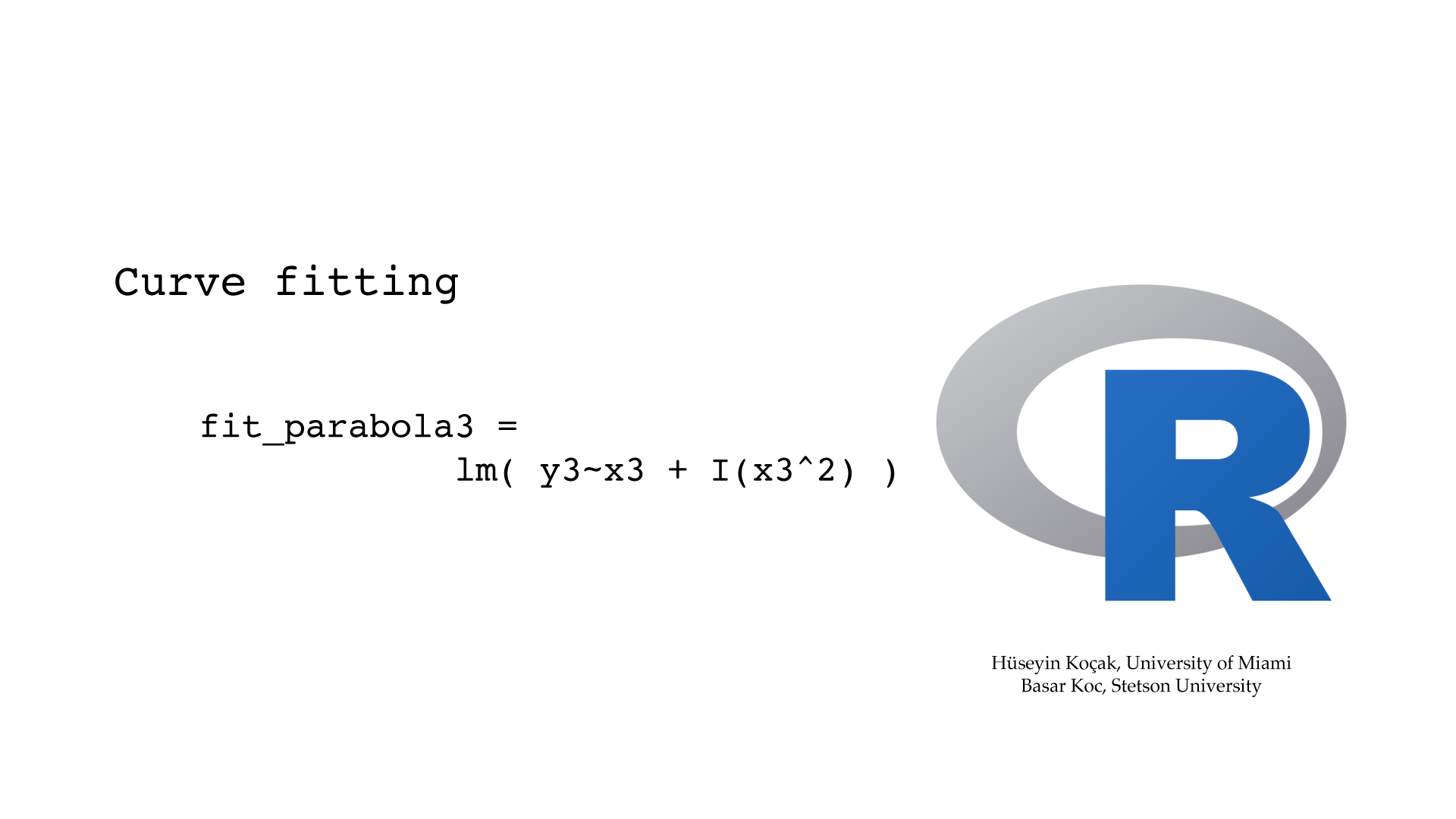```# ------------------------------------------------------------------
# File name: curve_fitting.R
#
# Linear Model function lm(y~x) computes the least square fit line to data points;
# y as a function of x. Type ?formula for help with legal formulas.
# lm() can also be used to fit nonlinear models where the parameters enter into model linearly.
#
# Version: 2.1
# Authors: H. Kocak, University of Miami and B. Koc, Stetson University
# References:
# https://www.r-project.org
# ------------------------------------------------------------------

# Fit a line to two data points; a good test of lm()
x2 = c(1, 2)
y2 = c(1.5, 2.5)

plot(x2, y2, main="Unique line through 2 points", xlim=c(0, 4), ylim = c(0, 4))

# Compute the least square line, save output in ls_fit_line
ls_fit_line = lm(y2 ~ x2)
print(ls_fit_line)

# Plot the least square line stored in ls_fit_line
abline(ls_fit_line, col="red")

# Print the vector containing intercept and slope the line
print(coef(ls_fit_line))

print(summary(ls_fit_line))

# Compute least-square line fit for 3 data points
x3 = c(1, 2, 3)
y3 = c(1.5, 2.5, 2.8)

plot(x3, y3, main="Best-fit line through 3 points", xlim=c(0, 4), ylim = c(0, 4))
fit3 = lm(y3 ~ x3)
abline(fit3, col = "red")
print(fit3)

print(summary(fit3))

# Compute the parabola through 3 data points

plot(x3, y3, main="Unique parabola through 3 points", xlim=c(0, 6), ylim = c(0, 6))
fit_parabola3 = lm(y3 ~ x3 + I(x3^2))
print(fit_parabola3 )
curve(coef(fit_parabola3) + coef(fit_parabola3)* x + coef(fit_parabola3)*x^2,
0, 6, col = "red", add=TRUE)

# Compute a best-fit parabola for 4 data points

x4 = c(1, 2, 3, 4)
y4 = c(1.5, 2.5, 2.8, 1.7)

plot(x4, y4, main="Best-fit parabola through 4 points", xlim=c(0, 6), ylim = c(0, 6))
fit_parabola4 = lm(y4 ~ x4 + I(x4^2))
print(fit_parabola4)
curve(coef(fit_parabola4) + coef(fit_parabola4)* x + coef(fit_parabola4)*x^2,
0, 6, col = "red", add=TRUE)

points(x4, predict(fit_parabola4), col = "green")

```

#### Beetle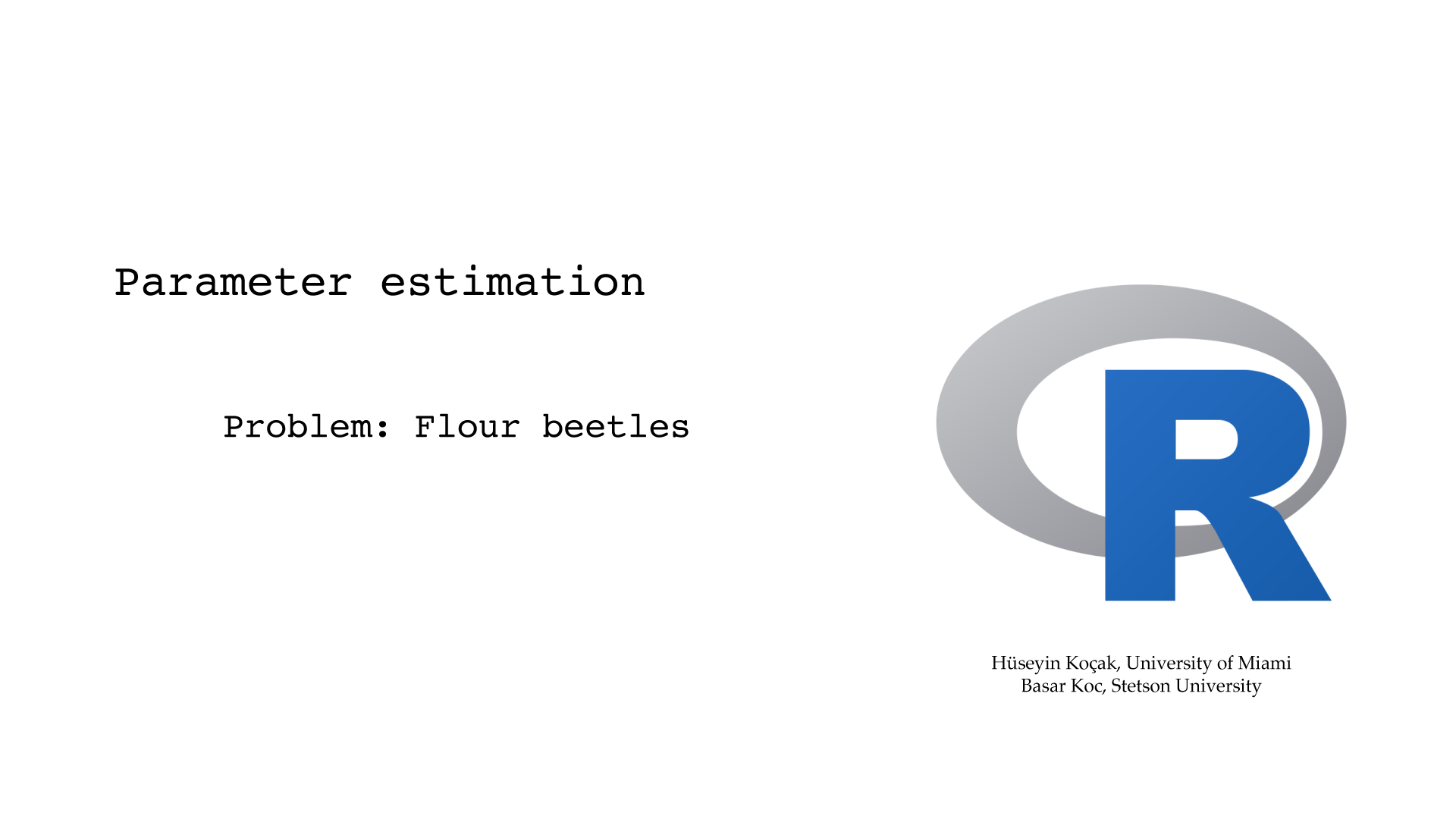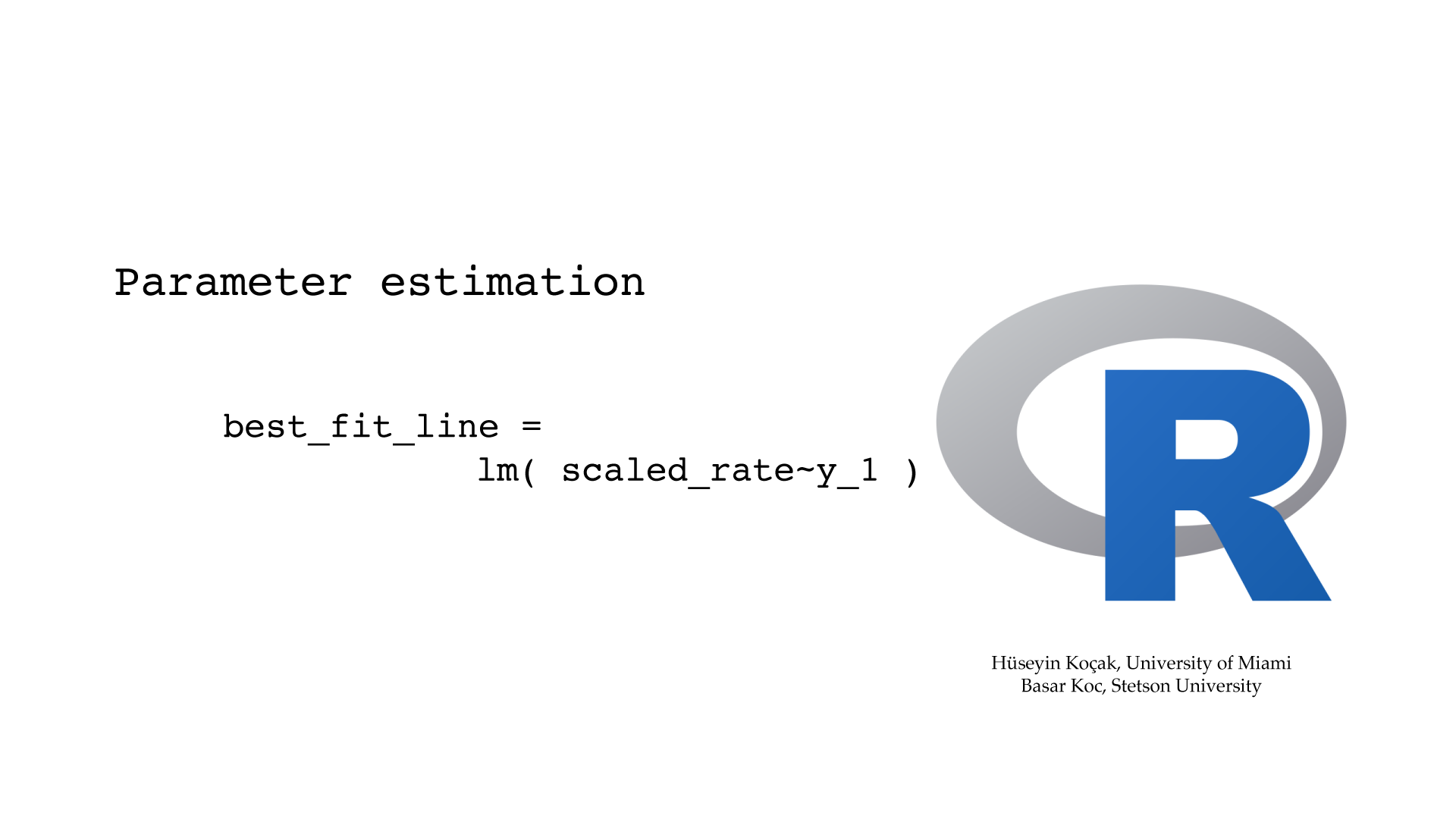```# ------------------------------------------------------------------
# File name: beetle_parameters.R
#
# Problem:
# Flour beetles with initial 112 individiuals are grown
# in a laboratory are counted daily for 15 days.
# Assuming that the beetle population grows according to the logistic differential
# equation (dy/dt) = ay + by^2, where y[t] is the population size at time t,
# estimate the values of the parameters a and b.
#
# Solution:
# Rewrite the differential equation as (dy/dt)/y = a + by so that r.h.s is linear in y
# Approximate the derivative with (y[t+h] - y[t])/h, here h=1,
# Compute  15  points, ((y[t+h] - y[t])/y[t], y[t]) and determine the best fit line.
# Intercept = a and slope = b.
#
# Version: 2.1
# Authors: H. Kocak, University of Miami and B. Koc, Stetson University
# References:
# https://www.r-project.org
# ------------------------------------------------------------------

# First, record the data and plot it daily count of beetles for 16 days

# Beetle count for 15 days
y = c(112, 152, 212, 258, 306, 309, 315, 310,
298, 290, 303, 295, 311, 308, 299, 309)

# Time in days
t = seq(0, length(y)-1)

plot(t, y, main = "Daily beetle counts", type = "o", xlab = "days", ylab = "beetles")

# Remove the last data point (why?)
y_1 = y[-length(y)]

# To pause between plots

# Create a rate vector and load it with computed rates
scaled_rate = 0

for(i in 1:(length(y)-1)) {
scaled_rate[i] = (y[i+1] - y[i]) / y[i]
}

#print(rate)

# Plot 15 points and compute best line for them
plot(y_1, scaled_rate, col = "red", main = "Least square fit to scaled rates")

best_fit_line = lm(scaled_rate~y_1)       #compute line using lm() and save
abline(best_fit_line)                     #add the graph of the line
print(best_fit_line)                      #print intercept and slope of line

# If you know statistics, you can try the following commands for more information
# print(summary(best_fit_line))
# plot(best_fit_line)

```

1. Error vs. step size in Euler: We showed in class that the global error bound in Euler's algorithm is proportional to the step size. Now, in PHASER solve the initial-value problem x' = x, x(0) = 1 to compute x(1) = e = 2.7182818284590452354, using Euler's algorithm with seven different step sizes. Using R, plot the errors against the step sizes. Next, use the lm function of R to determine the "best-fit line" to the data points. What is the proportionality constant of errors vs. step size?

2. Finding the parabola: Three data points on the plane are given:
(-1, 1.1), (0, 2.05), (1.8, -0.5).
Find the unique parabola y = a + bx + cx^2 passing through these three points.

3. Beetle model: Download the R file beetle_parameters.R from the Beetle episode and run (source) it. Read out the parameter values of a and b for the logistic differential equation y' = ay + by^2. Now, use these parameter values in the Cubic1D ODE differential equation in Phaser, with the appropriate initial condition, and print out the Xi values for 14 days.
Now write an R code that computes the averages of the data points and Phaser points and prints out the difference between the two averages.

4. Heating of a probe: A student held a temperature probe between her two fingers and recorded the following temperatures every 2 seconds:
Time     Temp
00     32.77
02     33.16
04     33.35
06     33.57
08     33.67
10     33.75
12     33.85
14     33.94
16     33.98
18     34.03
20     34.04
• Plot the temps as a function of time. Is the temp leveling off? Why?
• Plot the numerical approximation of the derivative from the data vs. the temperature. Notice that this graph looks almost linear. Now compute the least squares fit line to this graph and determine the equation of the line.
• What is the differential equation and the initial condition governing this experiment? Put this differential equation into Phaser and plot the solution. Does the Phaser plot resemble the experimental data?